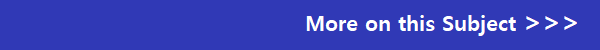## OCR GCSE Maths Past Papers

OCR GCSE Maths (9-1) (J560) past exam papers. Prior to 2017 OCR ran two syllabuses Mathematics A and Mathematics B. If you are not sure which syllabus you are studying or which exam tier (foundation or higher) you are sitting check with your teacher. You can download the OCR GCSE maths past papers and marking schemes by clicking on the links below.

OCR Maths GCSE (9-1) June 2022: Mathematics (J560)

OCR Maths GCSE (9-1) Foundation Papers

June 2022 Mathematics J560/01 Paper 1– Foundation

June 2022 Mathematics J560/02 Paper 2– Foundation

June 2022 Mathematics J560/03 Paper 3– Foundation

OCR Maths GCSE (9-1) Higher Papers

June 2022 Mathematics J560/04 Paper 4– Higher

June 2022 Mathematics J560/05 Paper 5– Higher

June 2022 Mathematics J560/06 Paper 6– Higher

OCR Maths GCSE (9-1) November 2021: Mathematics (J560)

OCR Maths GCSE (9-1) Foundation Papers

November 2021 Mathematics J560/01 Paper 1– Foundation

November 2021 Mathematics J560/02 Paper 2– Foundation

November 2021 Mathematics J560/03 Paper 3– Foundation

OCR Maths GCSE (9-1) Higher Papers

November 2021 Mathematics J560/04 Paper 4– Higher

November 2021 Mathematics J560/05 Paper 5– Higher

November 2021 Mathematics J560/06 Paper 6– Higher

OCR Maths GCSE (9-1) November 2020: Mathematics (J560)

OCR Maths GCSE (9-1) Foundation Papers

November 2020 Mathematics J560/01 Paper 1– Foundation

November 2020 Mathematics J560/02 Paper 2– Foundation

November 2020 Mathematics J560/03 Paper 3– Foundation

OCR Maths GCSE (9-1) Higher Papers

November 2020 Mathematics J560/04 Paper 4– Higher

November 2020 Mathematics J560/05 Paper 5– Higher

November 2020 Mathematics J560/06 Paper 6– Higher

OCR Maths GCSE (9-1) June 2019: Mathematics (J560)

OCR Maths GCSE (9-1) Foundation Papers

OCR Maths GCSE (9-1) Higher Papers

OCR Maths GCSE (9-1) November 2018: Mathematics (J560)

OCR Maths GCSE (9-1) Foundation Papers

OCR Maths GCSE (9-1) Higher Papers

OCR Maths GCSE (9-1) June 2018: Mathematics (J560)

OCR Maths GCSE (9-1) Foundation Papers

OCR Maths GCSE (9-1) Higher Papers

OCR Maths GCSE (9-1) June 2017: Mathematics (J560)

OCR Maths GCSE (9-1) Foundation Papers

OCR Maths GCSE (9-1) Higher PapersOCR Mathematics A    OCR Mathematics B

OCR Maths GCSE June 2016: Mathematics A (J562)

OCR Maths GCSE June 2015: Mathematics A (J562)

OCR Maths GCSE November 2014: Mathematics A (J562)

OCR Maths GCSE June 2014: Mathematics A (J562)

OCR Maths GCSE November 2013: Mathematics A (J562)

OCR GCSE Maths B

OCR Maths GCSE June 2016: Mathematics B (J567)

OCR Maths GCSE June 2015: Mathematics B (J567)

OCR Maths GCSE November 2014: Mathematics B (J567)

OCR Maths GCSE June 2014: Mathematics B (J567)

(Mathematics B: Paper 4 – Question 11 loose sheet)

OCR Maths GCSE November 2013: Mathematics B (J567)